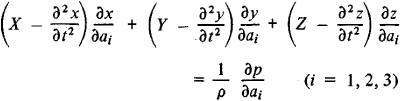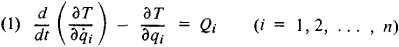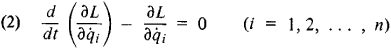# Lagrange Equations

The following article is from The Great Soviet Encyclopedia (1979). It might be outdated or ideologically biased.

## Lagrange Equations

(1) In fluid mechanics, the equations of motion of a fluid medium written in Lagrangian variables, which are the coordinates of particles of the medium. The law of motion of the particles of a medium is determined from the Lagrange equations in the form of time-dependent functions of the coordinates, and the paths, velocities, and accelerations of the particles are thereby found. Generally, this method of investigation proves to be quite complex, and thus other methods using Euler’s equations of fluid mechanics are used to solve most problems in fluid mechanics. The Lagrange equations are primarily used in the study of the oscillatory motion of a fluid.

The Lagrange equations are partial differential equations and have the formwhere t is time; x, y, and z are the coordinates of the particle; a1, a2, and a 3 are parameters that distinguish the particles from one another (for example, the initial coordinates of the particles); X, Y, and Z are the components of the external force; ρ is pressure; and ρ is density.

The solution of actual problems reduces to finding x, y, z, p, and ρ as functions of t and a1, a2, and a 3, when X, Y, and Z as well as the initial and boundary conditions are known. It is also necessary to use the equation of continuity (also in Lagrangian variables) and an equation of state in the form ρ = f(p)(for an incompressible fluid, ρ = const).

(2) In general mechanics, the Lagrange equations are equations used in the study of the motion of a mechanical system in which independent parameters, called generalized coordinates, are selected as the variables that determine the position of the system. These equations were first obtained by J. Lagrange in 1760.

The motion of a mechanical system can be studied either by using equations given directly by the second law of dynamics or by using general theorems obtained from the laws of dynamics. In the first method, it is necessary to solve a large number of equations, depending on the number of points and bodies in the system. These equations also contain additional unknowns in the form of superimposed constraints. As a result, enormous mathematical difficulties arise. The second method requires the use of different theorems for each case and thus for complex systems ultimately leads to the same difficulties.

For a large class of mechanical systems, the Lagrange equations provide a unique and sufficiently simple method of constructing equations of motion that is independent of the form (complexity) of the actual system. The chief advantage of the Lagrange equations is that their number is equal to the number of degrees of freedom of the system and is independent of the number of points and bodies in the system. For example, engines and machines consist of many bodies (components) and usually have one or two degrees of freedom. Consequently, the study of their motion requires the setting up of only one or two Lagrange equations. In addition, under ideal conditions, all unknown constraints are automatically excluded from the Lagrange equations. For these reasons the Lagrange equations are widely used in the solution of many problems in mechanics, in particular, problems dealing with the dynamics of engines and machines and problems in vibration theory, the theory of the gyroscope, and other areas. In addition, whenever only forces derivable from a potential act on a system, the Lagrange equations are reduced to a form that permits them to be used (with a corresponding generalization of the concepts) not only in mechanics but also in other branches of physics.

For holonomic systems, the Lagrange equations in the general case have the formwhere the qi are generalized coordinates whose number is equal to the number n of degrees of freedom of the system, the i are generalized velocities, the Qi are generalized forces, and T is the kinetic energy of the system expressed in terms of qi and i.

In order to set up the equations (1), it is necessary to find the expression for T and to calculate Qi from the given forces. After T is substituted on the left-hand sides, the equations (1) will contain the coordinates q, and their first and second derivatives with respect to time, that is, they will be second-order differential equations in qi. The functions qi,(t), that is, the law of motion of the system in generalized coordinates, can be found by integrating these equations and by determining the integration constants from the initial conditions.

When only forces derivable from a potential act on the system, the Lagrange equations take the formwhere L = T — Π is the Lagrangian function, with Π the potential energy of the system. These equations are also used in other branches of physics.

Equations (1) and (2) are also called the Lagrange equations of the second kind. The Lagrange equations of the first kind have the form of ordinary equations in Cartesian coordinates and instead of constraints contain undetermined multipliers proportional to them. These equations do not possess any special advantages and are rarely used; they are used primarily to find the constraints when the law of motion of the system is found by other methods, for example, by means of equations (1) or (2).

### REFERENCE

Kochin, N. E., I. A. Kibel’, and N. V. Roze. Teoreticheskaia gidrome-khanika, 6th ed., part 1. Moscow, 1963.

S. M. TARG

References in periodicals archive ?
The Lagrange equations of the second kind were used to find the motion equations.
Lagrange equations in the presence of holonomic constraints are of the form :
The mathematical modeling of inverted pendulum system is derived based on Lagrange equations. Lagrange equation uses Kinetic and potential energies of mass stored in the pendulum [1,2].
Dynamic equations of the system are obtained on the basis of Lagrange equations. The dynamic system has two degrees of freedom (i.e., the pitch and the plunge motions).
Dynamic equations for the motion of the mechanical system will be derived using the Lagrange equations [14, 16-18] for generalized coordinates [x.sub.1], [x.sub.2], and [alpha].
In order to solve the problem, we introduce the Lagrange equations for the motion of the mechanical system "crane boom B[O.sub.2]-load M" which is shown in Figure 1.
In this section, in order to verify the obtained dynamics model since the motion equations by Newton-Euler method are inhomogeneous unlike Lagrange equations, a desired path for the quadrotor flight is defined.
To integrate the planetary Lagrange equations, it is necessary to develop the second member of the Lagrange planetary equations as Fourier series with respect to the selected anomalies for each couple of planets.
The evolution equations of the Lagrangian supermechanical system [summation] are the following Lagrange equations:
The second edition of this guide to analyzing liquid crystal displays (LCDs) reflects advances in the field over the past decade, with new discussion of areas such as molecular reorientation, polarizers, biaxial films, vertically aligned LCDs, spherical trigonometry, and Lagrange equations. The book offers a self-contained treatment of the physics of optical components employed in LCDs, and explains how to design and analyze optical components and subsystems in LCD systems.

Site: Follow: Share:
Open / Close